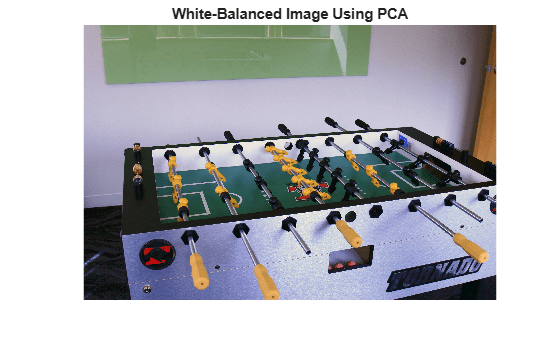# illumpca

Estimate illuminant using principal component analysis (PCA)

## Syntax

``illuminant = illumpca(A)``
``illuminant = illumpca(A,percentage)``
``illuminant = illumpca(___,'Mask',mask)``

## Description

example

````illuminant = illumpca(A)` estimates the illumination of the scene in RGB image `A` from large color differences using principal component analysis (PCA).```
````illuminant = illumpca(A,percentage)` estimates the illumination using the specified percentage of darkest and brightest pixels.```
````illuminant = illumpca(___,'Mask',mask)` estimates the illumination using only the pixels within the ROI defined by a binary mask.```

## Examples

collapse all

Open an image and display it. Specify an optional magnification to shrink the size of the displayed image.

```A = imread('foosball.jpg'); figure imshow(A,'InitialMagnification',25) title('Original Image')```Principal component analysis assumes that the RGB values are linear. However, the JPEG file format saves images in the gamma-corrected sRGB color space. Undo the gamma correction by using the `rgb2lin` function.

`A_lin = rgb2lin(A);`

Estimate the scene illumination from the darkest and brightest 3.5% of pixels (the default percentage). Because the input image is linear, the `illumpca` function returns the illuminant in the linear RGB color space,

`illuminant = illumpca(A_lin)`
```illuminant = 1×3 0.4075 0.5547 0.7254 ```

The third coefficient of `illuminant` is the largest, which is consistent with the blue tint of the image.

Correct colors by providing the estimated illuminant to the `chromadapt` function.

`B_lin = chromadapt(A_lin,illuminant,'ColorSpace','linear-rgb');`

To display the white-balanced image correctly on the screen, apply gamma correction by using the `lin2rgb` function.

`B = lin2rgb(B_lin);`

Display the corrected image, setting the optional magnification.

```figure imshow(B,'InitialMagnification',25) title('White-Balanced Image using Principal Component Analysis')```## Input Arguments

collapse all

RGB image, specified as an m-by-n-by-3 numeric array.

Data Types: `single` | `double` | `uint8` | `uint16`

Percentage of pixels to retain for the illuminant estimation, specified as a numeric scalar in the range (0, 50].

Data Types: `single` | `double` | `int8` | `int16` | `int32` | `int64` | `uint8` | `uint16` | `uint32` | `uint64`

Image mask, specified as an m-by-n logical or numeric matrix. The mask indicates which pixels of the input image `A` to use when estimating the illuminant. The computation excludes pixels in `A` that correspond to a mask value of 0. By default, the mask has all 1s, and all pixels in `A` are included in the estimation.

Data Types: `single` | `double` | `int8` | `int16` | `int32` | `int64` | `uint8` | `uint16` | `uint32` | `uint64` | `logical`

## Output Arguments

collapse all

Estimate of scene illumination, returned as a 3-element numeric row vector. The three elements correspond to the red, green, and blue values of the illuminant.

Data Types: `double`

## Tips

• The algorithm assumes uniform illumination and linear RGB values. If you are working with nonlinear sRGB or Adobe RGB images, use the `rgb2lin` function to undo the gamma correction before using `illumpca`. Also, make sure to convert the chromatically adapted image back to sRGB or Adobe RGB by using the `lin2rgb` function.

## Algorithms

Pixel colors are represented as vectors in the RGB color space. The algorithm orders colors according to the brightness, or norm, of their projection on the average color in the image. The algorithm retains only the darkest and brightest colors, according to this ordering. Principal component analysis (PCA) is then performed on the subset of colors. The first component of PCA indicates the illuminant estimate.

 Cheng, Dongliang, Dilip K. Prasad, and Michael S. Brown. "Illuminant Estimation for Color Constancy: Why spatial-domain methods work and the role of the color distribution." Journal of the Optical Society of America A. Vol. 31, Number 5, 2014, pp. 1049–1058.